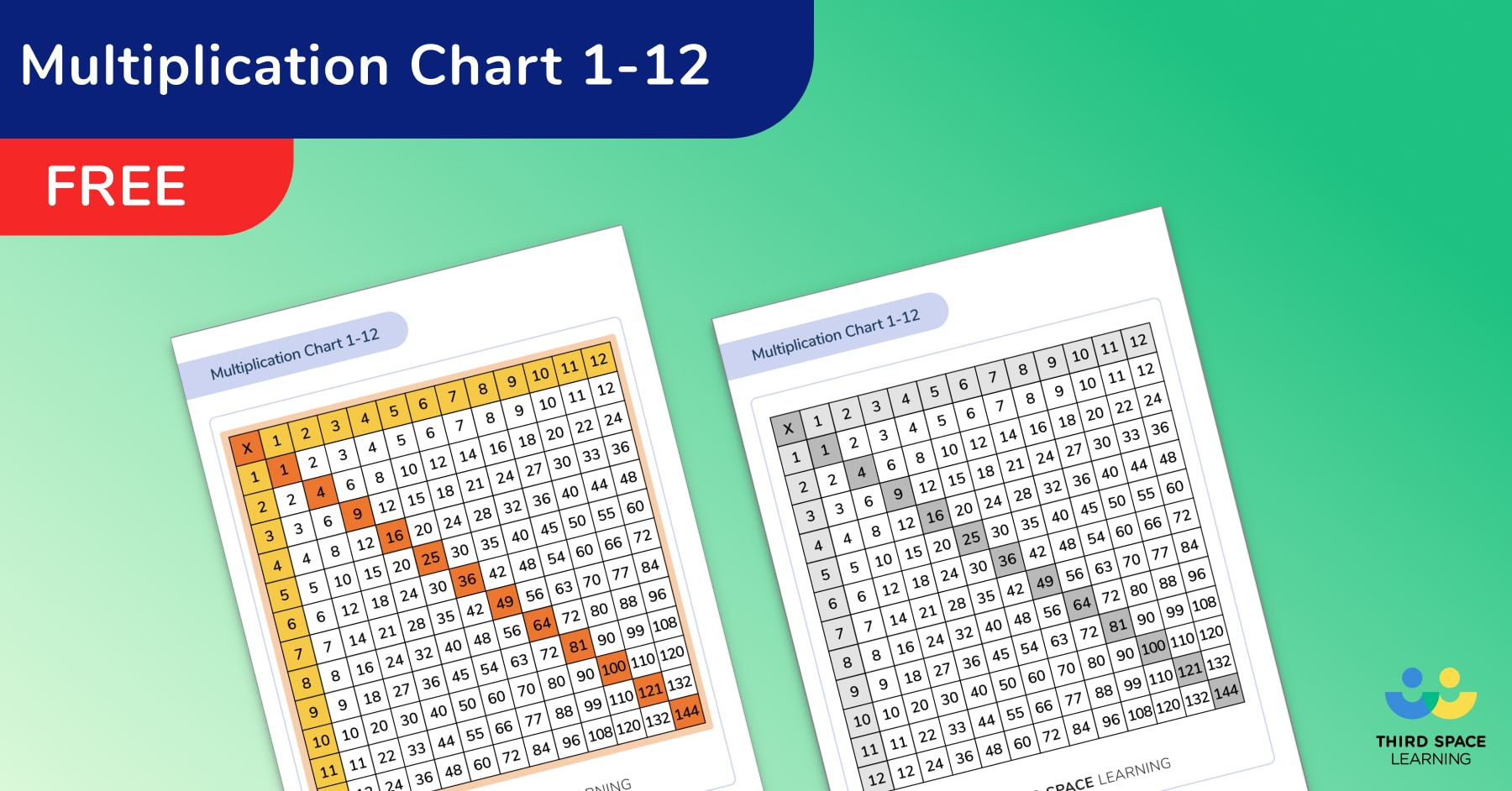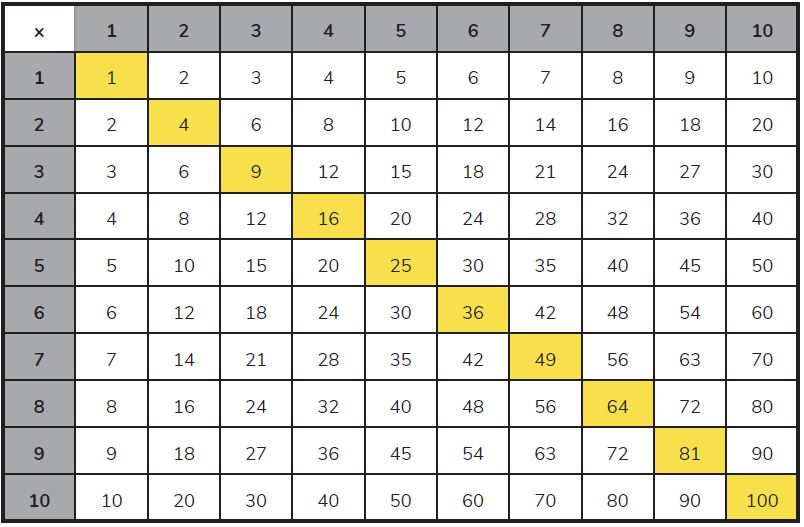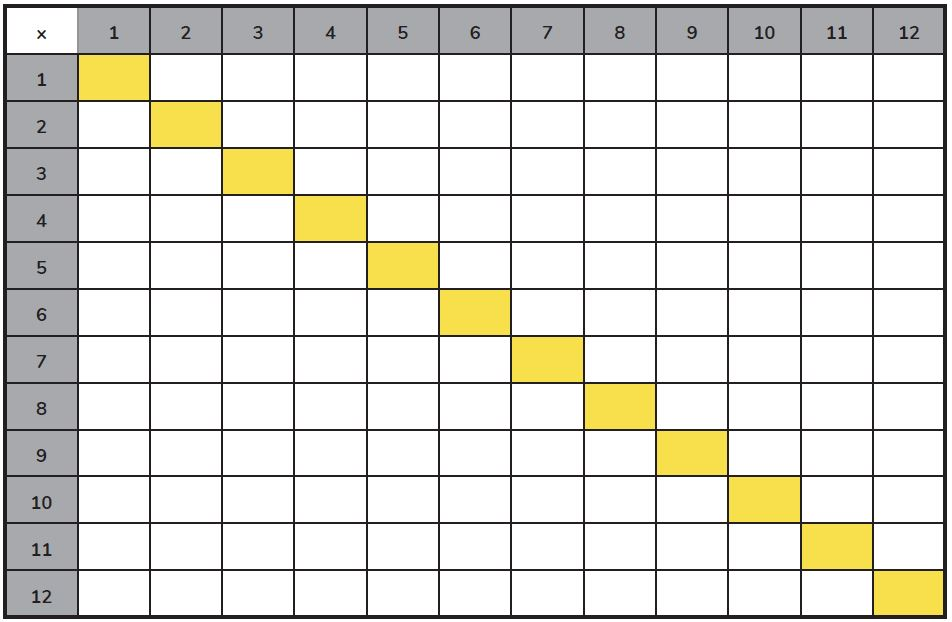Math teaching support you can trustone-on-one tutoring sessionsschools supported

[FREE] Themed Math Problems: Fall Term (Sep – Dec)

5th grade math problems and answers that link to key dates in the Fall Term, including Christmas!

# Why And How Every Teacher Should Be Using A Multiplication Chart In Elementary Math Lessons

Here we show you how to make the most of the multiplication chart: one of the most versatile numeracy tools you have to support the teaching and learning of times tables in elementary school.

Some call it a multiplication grid or a multiplication chart, but whatever your preference, it is our mission to encourage you to familiarize yourself with all varieties of the multiplication chart and incorporate it in some form or another in all your future multiplication facts teaching.

### What is a multiplication chart?

A multiplication chart is a visual, at-a-glance, way of displaying all the multiplication tables at once. Generally, you’ll use a 12 x 12 times tables grid or a 10 x 10 times tables grid (up to 100 grid).

There are several alternative names for a multiplication chart, such as a times table grid, multiplication tables grid, multiplication grid, times tables grid or times table square, and sometimes even a 100 square, or a hundred square. All of these are variations on what we shall mostly refer to as a multiplication chart.

A multiplication chart is an incredibly versatile and effective learning resource, and, unlike teachers’ favorites (which we love too) Jack Harmann and Multiplication.com, your multiplication chart still works when the internet goes down or you can’t get access to any devices. All you need is some printed copies stashed away in your drawer and a completed chart in each of your students’ workbooks.Multiplication Chart 1-12

A multiplication chart showing the times tables from 1 – 12Download Free Now!

Here are some examples of a multiplication chart:

12 Multiplication Chart

100 Square / 100 Multiplication ChartThe original multiplication chart up to 100, also called a 100 square or a hundred square.

They’re simple but effective, and extremely versatile. As is a blank multiplication chart and a blank 100 square.

### Blank Multiplication ChartStudents can fill in a blank multiplication square or 12 multiplication chart like this one over time,learning multiplication as they go.

Blank 100 Square

### Why you should use multiplication charts

Multiplication facts are the building blocks of so many elements of mathematics, and if you believe in the systematic teaching of these facts, then you should believe in the value of the humble multiplication chart.

Treasuring multiplication facts and making mastery of multiplication a central feature of your school’s culture is a key way to get ahead of the times tables game.

### How to use a multiplication chart

Whether you use a pre completed multiplication chart or a blank one will depend on your needs in class, but they are versatile enough to use across all age groups. Here are a few ideas to use your multiplication charts to practice your students’ multiplication skills.

#### Using a multiplication chart in 3rd grade

It can be an excellent tool to introduce students in 3rd grade to multiplication and division facts, showing them the relationship between these two operations.

For example that 2 x 3 = 6 and that 6 ÷ 2 = 3.

#### Using a multiplication chart in 4th or 5th grade

At 4th grade and above, students will be reinforcing what was learned in lower grades – so a multiplication chart is perfect for those students still lagging a little ways behind.

Due to their pictorial nature, they are also excellent tools to help kids with special educational needs.

#### Using a multiplication chart in 6th grade

For 6th Grade, when introducing the potentially confusing subject of lowest common multiples and highest common factors, multiplication charts are a simple pictorial representation of what is meant by a multiple or factor.

For example, looking at the 7 times table will highlight what numbers are factors of 7. By then showing students the multiplication calculation 7 x 9 = 63 this then shows them that 9 and 7 are factors of 63.

### Blank multiplication grid ideas

• You could start with a blank multiplication table chart up to 100 – either with or without the factors marked on the far left column and top row – and have children fill them in themselves.
• Or, you could launch straight into having your students fill in their multiplication chart up to 12 x 12 from scratch.
• Whether you choose to use a multiplication chart up to 100 or a one up to 12 x 12, the ‘filling in’ could happen at school, or it could be part of student’s home learning, filling in the relevant column or row with different numbers depending on the times tables being focused on each week.
• Use the same format of a square of related facts to build knowledge of square numbers or decimals.

Each child’s grid could then be seen as a visual, numerical learning journal growing over time as their attention grows from one multiplication fact to the next.

### Multiplication chart activities to challenge students

If you really wish to challenge your students, you could have randomized products inside cells on a multiplication chart. This means that students have to work out which column and row headings to place based on their multiplication fact knowledge – or knowledge of multiplication fact rules.

An example of this is how all numbers in the five times table end with the digits 5 or 0, or that the sum of the digits in the nine times table is either 9 or in the case of 9 x 11 = 99…. 9 + 9 = 18 (a multiple of nine itself at least, which – again – if the sum of the digits is added together finally makes 9 too).

The true problem solvers will quickly catch on that they can copy the headings for the columns and rows using the numbers in the one times table column and row respectively.

You can test how much they’ve mastered their multiplication knowledge by asking for a reasoned explanation for their hack or shortcut!

### Multiplication chart activities to develop reasoning skills

Reasoning from derived facts and the ability to incorporate multiplying and dividing by 10, 100 and 1,000 are key mental strategies that can improve children’s speed and confidence.

The grid below demonstrates how they can be used as the perfect pro forma to positively impact children’s multiplication agility – especially when used as a multiplication chart race! The example below includes multiplying decimals so it is a great challenge for 5th graders. It can be adapted to serve the learning needs of your students.

To extend the format further, you could also have a mixture of filled in product cells and empty column / row heading cells to challenge children to work out what the original factors were in your times tables worksheet…

### Multiplication chart games

Here are two multiplication games you can play with your class to make multiplication practice more fun.

#### Multiplication chart game 1: Grid Dice

For our multiplication chart dice game, as you might have already guessed, you’ll need a blank black and white printable multiplication table with grids up to 12 x 12 (the yellow section will be explained!) and pairs of six-sided dice.

Instructions:

Children can play this game in pairs or as a table group.

• Each child should have a pen or pencil of their own different color, and one die each.
• To begin with the children can roll one die to find the first factor and then the same die to choose the second factor. So, if they rolled 2 and then 6, they would fill in the 2 x 6 cell and the 6 x 2 cell – a good time to demonstrate multiplication staying true to the commutative law.
• Once they’ve filled in up to 6 x 6 – the purpose of the yellow cells/box within the grid – they can start rolling both dice each time it’s their turn.
• If they roll a multiplication fact that’s already been filled in, they miss a go.
• Once the grid is entirely filled in, the children count up how many cells they’ve filled correctly (using one of our printable multiplication charts up to 12 x 12 to check their answers).

(You can differentiate by setting up the game using a blank times multiplication chart up to 100, again checking at the end using a printable multiplication chart up to 100.)

#### Multiplication chart game 2: Chart Races

Multiplication Chart Races are simple enough…

Start with columns and rows headed in numerical order. Children race to fill in all the missing numbers on their blank multiplication chart as quickly as possible. Encourage children to note down the time taken to allow them to see if they can improve on their time during future races.

Again, you can differentiate or show progression by using both multiplication charts up to 100 and grids up to 12 x 12.

You could then move your students on to races with the numerical column and row headings inverted.

From that point onwards, it’s best to randomize the column and row headings on the children’s blank grids. Then, they act as multiplication flashcards and encourage active recall of math facts.

### How a multiplication chart can be used in other subjects

Since multiplication facts are the foundation stone for so many other topics like division or fractions, a multiplication chart up to 12 x 12 is actually a fantastic resource to help children in these other topics.

• Without the cognitive load of working out their multiplication facts, reference to their multiplication chart can help a child access reasoning activities that would otherwise be beyond him.
• Having a multiplication chart could be the difference between a child being able to simplify a fraction or not.
• It also allows a child to choose an effective common denominator rather than being left frustrated in their fractions learning.
• Laminated and used as a poster or just a desk resource, the multiplication chart will act as a reminder to all students of what they’ve learned and what is still outstanding.

### How Third Space Learning uses multiplication charts

In the one to one tuition sessions, tutors are working to develop student independence and knowledge of known multiplication facts wherever possible. For this reason, keeping a multiplication chart in our toolkit of learning aids that math tutors use is just for those students who need the extra support.

A chart can be dragged onto a slide at the tutor’s discretion to support a student in getting to the answer using the strategy they’ve identified – whether they’re multiplying or dividing.

This is especially useful once students begin learning the long multiplication method and the long division method and we need to be wary of the cognitive load that remembering multiplication facts on top of the new method may be putting on them.

The virtual multiplication charts used by students in their online math lessons work much like a mini-whiteboard or laminated grid.

Students can make notes on the grid, personalize it, make it work for them in their learning, leave it to one side (by hiding it) and then return to it with the same annotations which often prove useful if it’s returned to later on in the lesson.

Do you have students who need extra support in math?
Give your students more opportunities to consolidate learning and practice skills through personalized math tutoring with their own dedicated online math tutor.

Each student receives differentiated instruction designed to close their individual learning gaps, and scaffolded learning ensures every student learns at the right pace. Lessons are aligned with your state’s standards and assessments, plus you’ll receive regular reports every step of the way.

Personalized one-on-one math tutoring programs are available for:

The content in this article was originally written by Alexander Athienitis at Third Space Learning and has since been revised and adapted for US schools by elementary math teacher Christi Kulesza.##### Alexander Athienitis
ThirdSpaceLearning
Social Media Manager
Alexander was most recently a Year 6 teacher in Tower Hamlets. He is working on developing the Maths Hub and sharing the power of 1-to-1 interventions via Third Space Learning's social media channels.
x#### Multiplication Chart 1-12

A multiplication chart showing the times tables from 1 – 12

#### Multiplication Chart 1-12A multiplication chart showing the times tables from 1 – 12